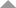Contact:
Niki Thiessen
[email protected]

Course Time:

• Tuesdays and Thursdays
Please note, this class meets twice per week.

Course Prerequisites:

• Students need to have completed Middle School Math 6/7
or an equivalent.
• Students scoring a B or better in a prerequisite class taken at WHCT
are not required to take a placement test for the next level class.
• All other students must pass a short placement test prior to registration.

Course Text:

• Prealgebra (4th edition)  -by Tom Carson
ISBN-  0321756959

Course Description:

Prealgebra is an introduction to Algebra.
It covers topics such as whole numbers, integers, solving equations and problem solving, fractions, mixed numbers,
decimals, ratios, proportion, percent, graphing, geometry and measurement, exponents, and polynomials.

Course Class Time:

• Go over previous homework.
• Learn new concepts.
• Practice and take questions.

Course Homework Requirements:

• There are 5 homework assignments each week. Most assignments can be completed in less than 1 hour.
• Each Tuesday students will receive a summary of that week’s assignments, including page numbers and the specific problems to be completed.
• On classroom days students will receive fill-in-the-blank notes to follow along with the lecture and work practice problems for the new concepts.
• There will be two Math XL assignments per week (normally Mondays and Wednesdays).
• Students are expected to check answers to odd-numbered problems at home.
• Questions regarding even-numbered problems are addressed at the beginning of class.

Testing:

• Tests will be administered in-class.

• 40%  homework and practice tests
• 40%  tests
• 20%  math XL

• 10%  midterm

• 10%  final

Course Supplies:

• textbook
• binder w. dividers  -labeled Notes, Homework, Resources, and Tests
• loose-leaf paper
• loose-leaf graph paper
• pencils / blue and red pens / highlighter
• calculator such as TI- 30X IIS   (\$15 or less) (by third quarter)
• ruler (4th quarter)